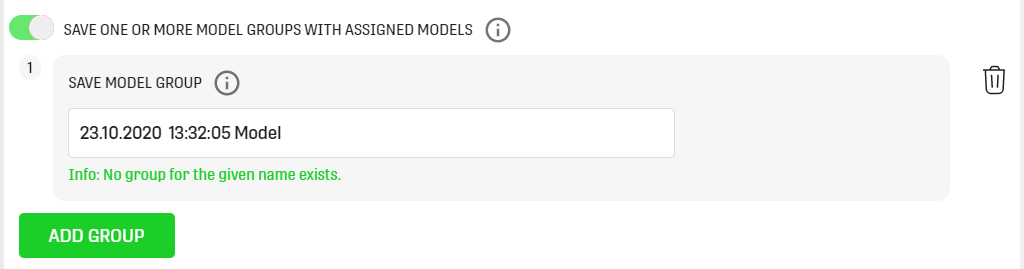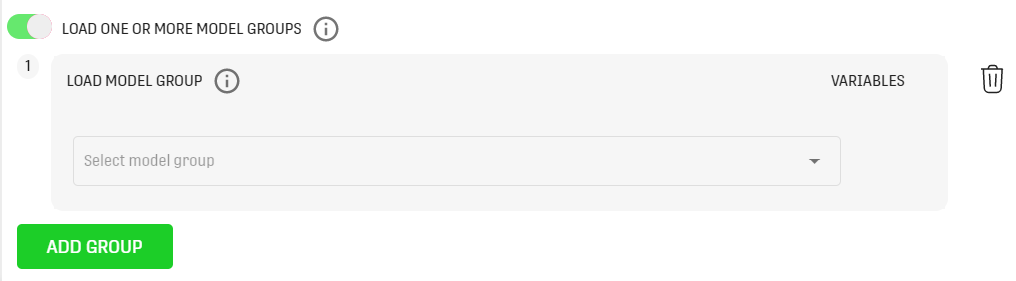# Motivation

With ONE DATA there are many ways to process data within a Workflow using different Processors. But sometimes, it is necessary or easier to use custom computation methods. A Python script, for instance, is a good way to customize the processing of data, due to the fact that Python is a highly flexible programming language with many open source libraries.

Since Python scripts are a very useful tool, it is possible to include them in Workflows with the ONE DATA Python Processors. In this article, the one we are focusing on is the Python Script Data Generator Processor.

# Overview

With the Python Script Data Generator Processor it is possible to generate data, either datasets or graphs and plots with a custom Python script. There are already some important libraries included, that could be necessary to write scripts for data science tasks. For further information on what packages are preinstalled, check the info on the left corner of the input box.To interact with ONE DATA resources from within the Python script, for example loading Models, accessing variables or specifying the output of the Processor, it is necessary to use the ONE DATA Python Framework.

`For advanced usage of the ONE DATA Python Processors, it can be really helpful to have a deeper look into the framework. This article gives a small insight to it, but does not cover the framework in depth`

# Input

The Processor takes Python code as input, that can be inserted in the top part of the configuration. When a new Python Script Data Generator is created, the "Python Script" field already contains some sample code, that provides basic code for creating a new dataset and its corresponding plot.# Configuration

The Processor configuration gives some additional options on how the output data should be processed and some definitions for the script execution itself. It is also possible to load ONE DATA  Python Models and use them in the script. All options are described more in-depth in the following sections.

##Timeout for Script Execution

This is the time in seconds that ONE DATA waits for the script execution and the returning of the results of the script. The time starts when the Processor submits the Python script and the data to the Python Service of ONE DATA. If the timeout is exceeds, the calculation will be interrupted.

The default value is 300 seconds.

## Generate Empty Dataset Output

This option defines if the Processor should generate an empty dataset after the execution of the script. This can be very useful, for example when the script is only used to generate a plot and no respective result dataset. This option can then be activated to prevent a Processor execution error, because by default, the Processor requires an output dataset.

## Manual TI

With this configuration option it is possible to specify what scale and representation type the columns of the output dataset have in order to provide the correct type inference in ONE DATA.

Possible scale types: nominal, interval, ordinal, ratio. Further information on scale types can be found here.

Possible representation types: string, int, double, datetime, numeric

If it is not possible to convert the values of a column to the specified representation type, the Processor will take the type that fits best for their representation. If types still do not fit the purpose, it is recommended to use the Data type Conversion Processor.

## Load One or More ModelsThe first dropdown is used to select an existing Python Model from the current project. Its also possible to specify which version of the Model should be loaded.

With the "Open Model" button you can access the Model view for the selected one directly from the Processor.

To use it in the script itself, a selected Model can be stored in a variable like so:

`model = od_models["model_name"]`

## Save One or More ModelsThis configuration option is used to save a generated Python Model to the project, or adds a new version to a existing one.

It has three options:

• Create New Model: Creates a new Model, with the name specified in the textbox below. The name needs to be unique within a Domain.
• Add New Model Version: Adds a new version to an already existing Model which can be selected below.
• Create Or Add Version: With this option the Processor either adds a new version to the given Model, or creates a new one if the Model does not exist yet.

`Note that, a Model needs to have a unique name within a Domain in ONE DATA.`

## Save One or More Model Groups With Assigned ModelsWith this option, you can save a Model Group created by Python within ONE DATA. All Model Groups added in the Python script must be configured in here, otherwise they will not be saved to the ONE DATA environment. To save a Model assigned to a Model Group stored in a variable Model under the name "my_model" and the Model Group name \"my_model_group\" use the following statement in the script:

`od_output.add_model("my_model", model, "my_model_group")`

`Note that, a Model Group needs to have a unique name within a Domain in ONE DATA.`

## Load One or More Model GroupsBy using this option, it is possible to load Model Groups for Python execution. Models of all loaded Model Groups will be accessible in the Python code in the dictionary: od_models

To load a Model named "my_model" and store it in a variable Model use the following statement:

`model = od_models["my_model"]`

# Output

The Python Script Data Generator Processor has several output types that can be defined within the script.

## Datasets

To pass a dataset as output to ONE DATA, the following method is used:

`od_output.add_data("output", dataset)`

A new dataset can be created in one of two ways:

• 2D matrix representation of data (list of rows where each row is a list of column values) and list of column names

```from onelogic.odpf.common import ODDataset
from datetime import datetime

dataset = ODDataset([[1, 2.0, "test", datetime.now()],
[2, 3.0, "sample", datetime.now()]],
["int_col", "double_col", "str_col", "timestamp_col"])```

• Pandas `DataFrame`

```from onelogic.odpf.common import ODDataset
from datetime import datetime
from pandas import DataFrame

d = {'int_col': [1, 2], 'double_col': [2.0, 3.0], 'str_col': ['test', 'sample'],
'timestamp_col': [datetime.now(), datetime.now()]}
dataset = ODDataset(DataFrame(data=d))```

Current restrictions:

• If content is passed as 2D matrix, column names must be specified and have the same size as each row.
• Data types in columns must be of supported type.

## Models

Like mentioned above in the configuration section, it is also possible to save Python Models to the project from within the script. This can be achieved like this:

`od_output.add_model("model_name", model_data)`

`Note that the "model_name" here has to exactly match the Model name specified in the Processor configuration.`

## Images

It is also possible to save plots and graphs generated within the script (for example with Pandas) as image to the "Image List" of the Processor. This can be done using the following method:

`od_output.add_image(image_name, image_type, image_data)`

where

• image_name is the name under which the image will be available in the Processor
• image_type is the type of the created image (either ImageType.PNG or ImageType.JPG)
• image_data is the image itself, either as byte array or a matplotlibs's Figure

# Example

In this example we want to generate a dataset that holds values for a specific timestamp, and also plot of the results as bar chart.

## Example Script

```from onelogic.odpf import ImageType
from onelogic.odpf.common import ODDataset
import datetime as dt
import pandas as pd
import time

example_values_y = [10, 20, 30, 40, 50]
example_values_x = []
example_values_time = []

for value in example_values_y:
example_values_x.append(value * 2)
current_time = dt.datetime.now()
example_values_time.append(current_time)

# create a plot using a pandas data frame
df = pd.DataFrame({'x': example_values_x, 'y': example_values_y, 'timestamp': example_values_time})
ax = df.plot.bar(x='x', y='y', rot=0)

# add image to our output - image will be available in Processor's result under the registered key

# publish the output

Within the script we have a for loop that takes every value of "example_values_y", doubles it and then saves the result to a new list. Additionaly it saves the timestamp of the computation to a list.

Then we create a Pandas dataframe from the three lists, and then use it to generate a simple bar chart.

In the end, we save the chart as JPG image and define the dataframe as output of the Processor.

## Workflow##In this example configuration we use the default timeout and we specify the types just for the "y" column. We won't load or save Models.

## Result

The plot image can be found within the "Image List" tab of the ProcessorAnd our Result Table looks as follows# Related Articles

Hands-On: Python Processors

Python Single Script Input Processor

Python Script Dual Input Processor

ONE DATA Python Framework